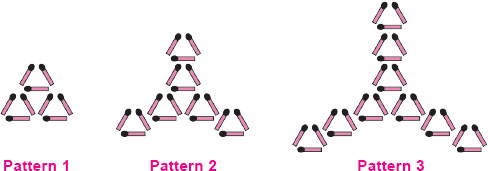# User ForumSubject :IMO    Class : Class 1

The sum of the number of matchsticks in Pattern 2 and Pattern 4 is _____.A 36
B 54
C 27
D 45

Class : Class 5
exactly

## Ans 2:

Class : Class 4
you have to find the pattern 4 yourself

Class : Class 5
exactly

## Ans 4: (Master Answer)

Class : Class 1
The correct answer is B.

## Ans 5:

Class : Class 5
Where is pattern 4

Class : Class 3

## Ans 7:

Class : Class 4
in the 1st figure 3 matchsticks make 1 whole triangle 3 triangles are there in 1st figure means 9 matchsticks in total. In 2nd figure 6 triangles are there means 18 matchsticks in total. In 3rd figure 9 triangles are there means 27 matchsticks in total. Now the series is 9, 18, 27 you are increasing by 9. So 27 plus 9 is 36. You got pattern 4 so add 18 and 36 which is 54